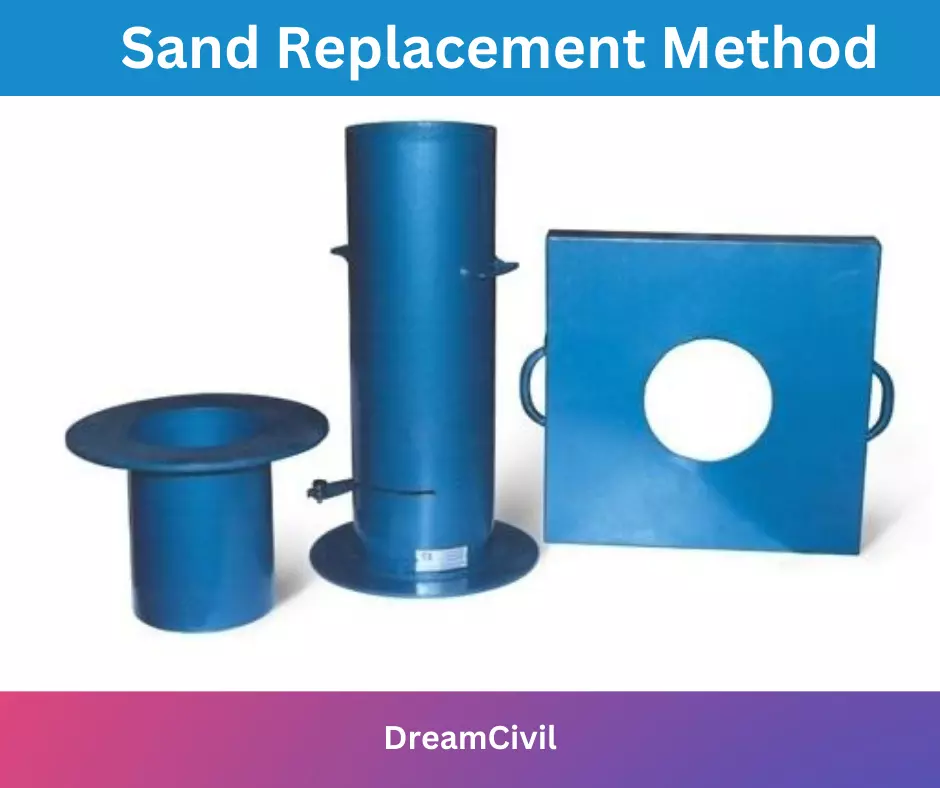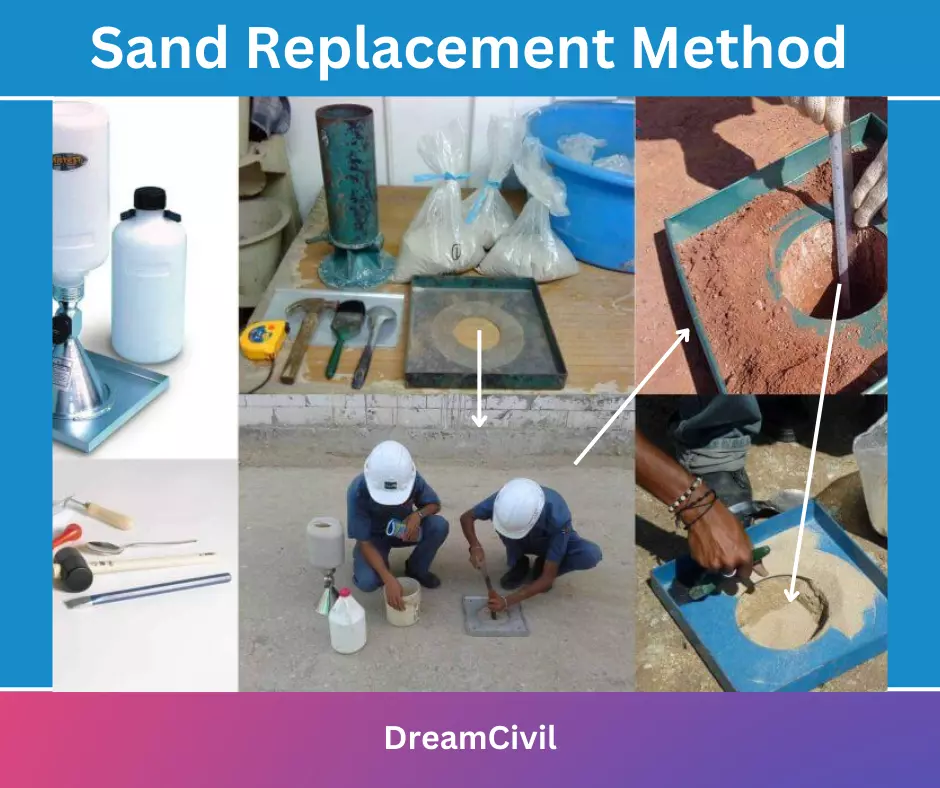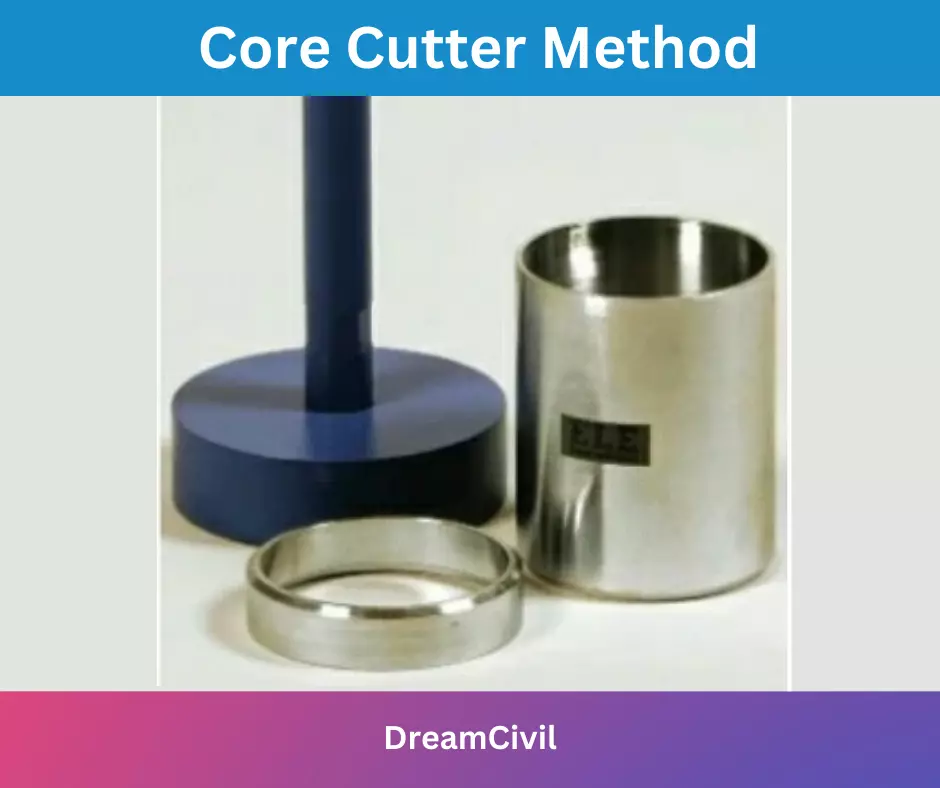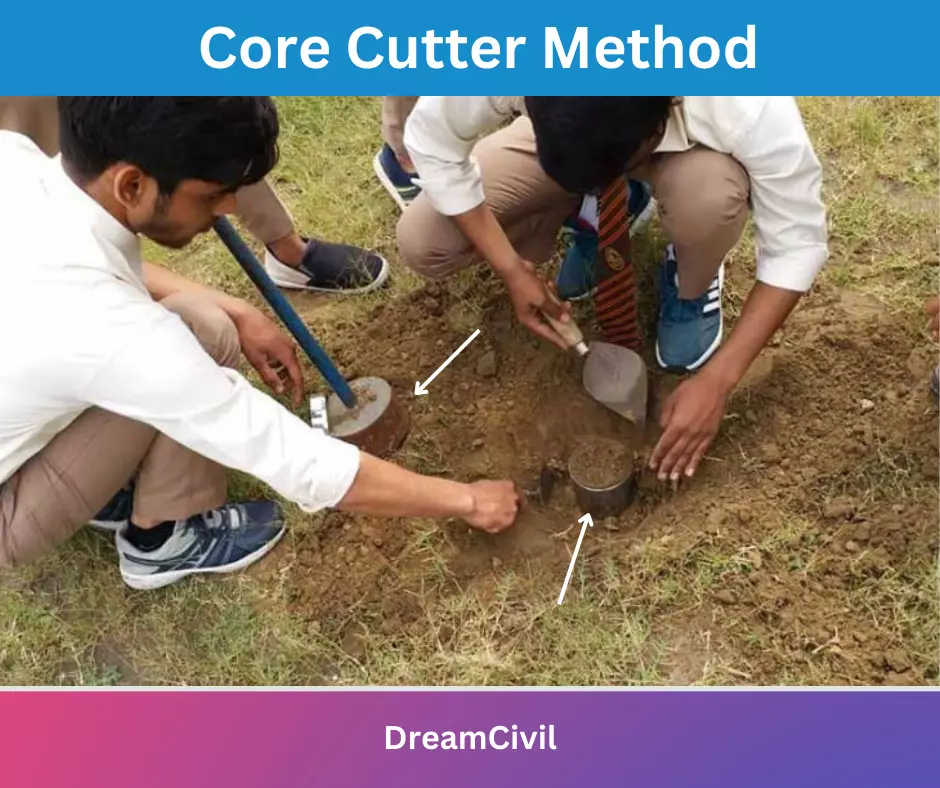Wednesday, October 4, 2023

# Methods of Compaction Control: Sand Replacement Method and Core Cutter Method

During the compaction of soils in the field, it is necessary to determine the dry density and water content of the compacted soil. More the dry density and water content, the more the shear strength and stability of the soil.
To check the dry density of soil, wet/ bulk density, and water content are measured by sand replacement method for cohesion less soil and core cutter method for cohesive soil.
After calculating wet density and water content, dry density can be determined by

γd = γt /(1+w)

where,

γd = dry density

γt = wet/ bulk density = (weight of soil/total volume of soil mass)= W/V

w= water content= (weight of water/weight of soil solids)= Ww /Ws

Note: water content used in the field compaction is termed ‘Placement water content’ with values lower, higher, or equal to OMC(Optimum Moisture Content).

## 1. Methods of Compaction Control

There are two methods of compaction control; Sand Replacement Method and Core Cutter Method.

### A. Sand Replacement Method

#### i. Aim

To determine the dry density of a given soil sample (cohesion less soil).

#### ii. Apparatus

Sand bottle, Conical funnel, Sand, Square tray with a circular hole#### iii. Procedures

a. A square tray with a circular hole in it is placed in the level ground and pressed on the ground to make hole

b. A small quantity of excavated soil from the hole is weighed and its water content is measured.

c. Using a sand bottle with a conical funnel, the hole is filled with sand of known density.

d. Weight of sand filled in the hole can be measured using readings of weights calculated.

#### iv. Observations

weight of soil mass = W

weight of sand bottle before filling of sand = W1

weight of sand bottle after filling of sand = W2

weight of sand filling with conical funnel = W3

weight of sand filled in hole = W4

unit weight of sand=γ

volume of sand = V = W4

W4 = W1 – W2 – W3

water content =w

Calculations

we have,

bulk density(γt) = W/V

dry density (γd) = γt /(1+w)#### v. Result:

Hence, dry density is calculated using the sand replacement method.

Steps to calculate the dry density using the sand replacement method:

a. Calculate the weight of sand filled in the hole.

W4 = W1 – W2 – W3

b. Calculate the volume of sand.

V = W4

c. Calculate bulk density /wet density.

γt = W/V

d. Calculate dry density.

γd = γt /(1+w)

sometimes, bulk density could be separately used as   γd = γ /(1+w)

### B. Numerical

The following results were obtained from the sand replacement method:

mass of soil excavated from the hole;  4 kg

the water content of soil;  18%

mass of dry soil to fill a hole;  3.1 kg

mass of dry sand to fill the container;  5.8 kg

the volume of the container; is 4.2litres

Calculate the wet and dry densities of the soil, if the specific gravity of the particles is 2.68.

Solution;

Given;  weight of sand = 5.8 kg

volume of sand = 4.2 × 10-3 m3

weight of sand to fill hole = 3.1 kg

weight of soil excavated = 4 kg

water content (w) = 18%

The wet density of soil( γt ) =?

the dry density of soil (  γd)=?

now,

Density of sand =5.8/(4.2 × 10-3 ) = 1380 kg/m3

Volume of hole = 3.1/1380 = 2.25× 10-3 m3

γt =total weight of soil excavated/ volume of hole = 4/ 2.25× 10-3 = 1778 kg/m

and,

we have,

w = Ww /Ws = weight of water/ weight of soil solids

18/100 = Ww /Ws

or, We = 0.18 Ws

Also, We + Ws = 4

or, 0.18 Ws + Ws =4

thus, Ws = 3.39 kg

thus,

dry density (  γd) = weight of dry soil/volume of the hole

= 3.39/ 2.25× 10-3 = 1507 kg/m

### C. Core Cutter Method

#### i. Aim

To determine the dry density of a given soil sample (cohesive soil)

#### ii. Apparatus

A cylindrical core cutter, dolly#### iii. Procedures

Note: ( soil sample must be soft, fine-grained soils of soil surface exposed so that cutter can be easily driven in the ground)

a. A cylindrical core cutter is embedded in the ground at its full height and a dolly is placed over the blade to prevent its edges from damage while embedding.

b. cutter is taken out from the ground and filled with soil.

c. Extra soil(surplus soil) at the top of both ends of the cutter is trimmed.

d. Then, the weight of the cylinder with excavated soil is weighed.

e. Volume of soil can be determined by known dimensions of the cutter.

f. Water content of the soil can be determined by oven drying method.

g. Using the formula, dry density can be calculated.

#### iv. Observations and Calculations:

Here,

water content/ moisture content =w

weight of cutter = W1

weight of soil and cutter = W2

weight of soil = W2 -W1

volume of cutter = v

bulk density (γt) =(W2 -W1)/ V

Dry density ( γd = γt /(1+w)#### v. Result

Using the core cutter method, the dry density of the soil sample given is calculated.

Steps to calculate the dry density of soft soil using the core cutter method:

a. Calculate the weight of the cutter with excavated soil in it.

weight of cutter = W1

weight of soil and cutter = W2

b. Calculate the weight of the soil.

weight of soil = W2 -W1

c. Calculate the volume of soil.

The volume of soil = volume of cylindrical cutter

d. Calculate the bulk density.

bulk density (γt) =(W2 -W1)/ V

e. Calculate the dry density.

Dry density ( γd = γt /(1+w)

where water content=w = weight of water/weight of soil solids

### D. Numerical

1. The in-situ density of an embankment, compacted at a water content of 12% was found out using the core cutter method. The empty cutter weighed 1286gms and the cutter with filled soil weighed 3195gms. The volume of the cutter was 1000cm3. Determine the bulk density and dry density of the barrier.

Solution;

Given; water content = 12% =0.12

weight of empty cutter = 1286gms

weight of cutter + soil = 3195gms

volume of cutter = V = 1000cm3

the dry density of soil (  γd)=?

bulk density of soil( γ) =?

Now,

weight of soil in cutter= W= 3195 – 1286 = 1909gms

volume of soil = volume of cutter=V

Here, bulk density = weight of soil/ volume of soil

= W/V = 1909/1000 = 1.909 gm/cm3

dry density, γd = γ /(1+w)

= 1.909/(1+0.12)

= 1.7 gm/cc

2. A 1000cc core cutter weighing 9467.8gm was used to find out the in-situ weight of an embankment. The weight of the core cutter filled with soil was found to be 2770.6gms. A sample has a water content of 10.45%. Determine the bulk density and dry unit weight of the dam.

Solution;

Given;  volume of cutter = 1000cc

Weight of empty cutter = 946.8gms

Weight of core cutter filled with soil = 2770.6gms

Water content of soil = 10.145% = 0.1045

Bulk density =?

Dry density =?

Now,

Weight of soil in cutter= 2770.6 – 946.8 =W

W= 1823.8gms

Weight of soil solid= W/(1+w)

= 1823.8/(1+0.1045) = 1651.2gms

Bulk unit weight = 1823.8/1000 = 1.82gm/cc

Dry unit weight= 1651.2/1000 = 1.65gm/cc

Hope you got an idea of methods of compaction control.

 Read Also: Design Considerations For Fire SafetyHe is a founder and lead author of Dream Civil International and his civil engineering research articles has been taken as source by world's top news and educational sites like USA Today, Time, The richest, Wikipedia, etc.
Latest Articles